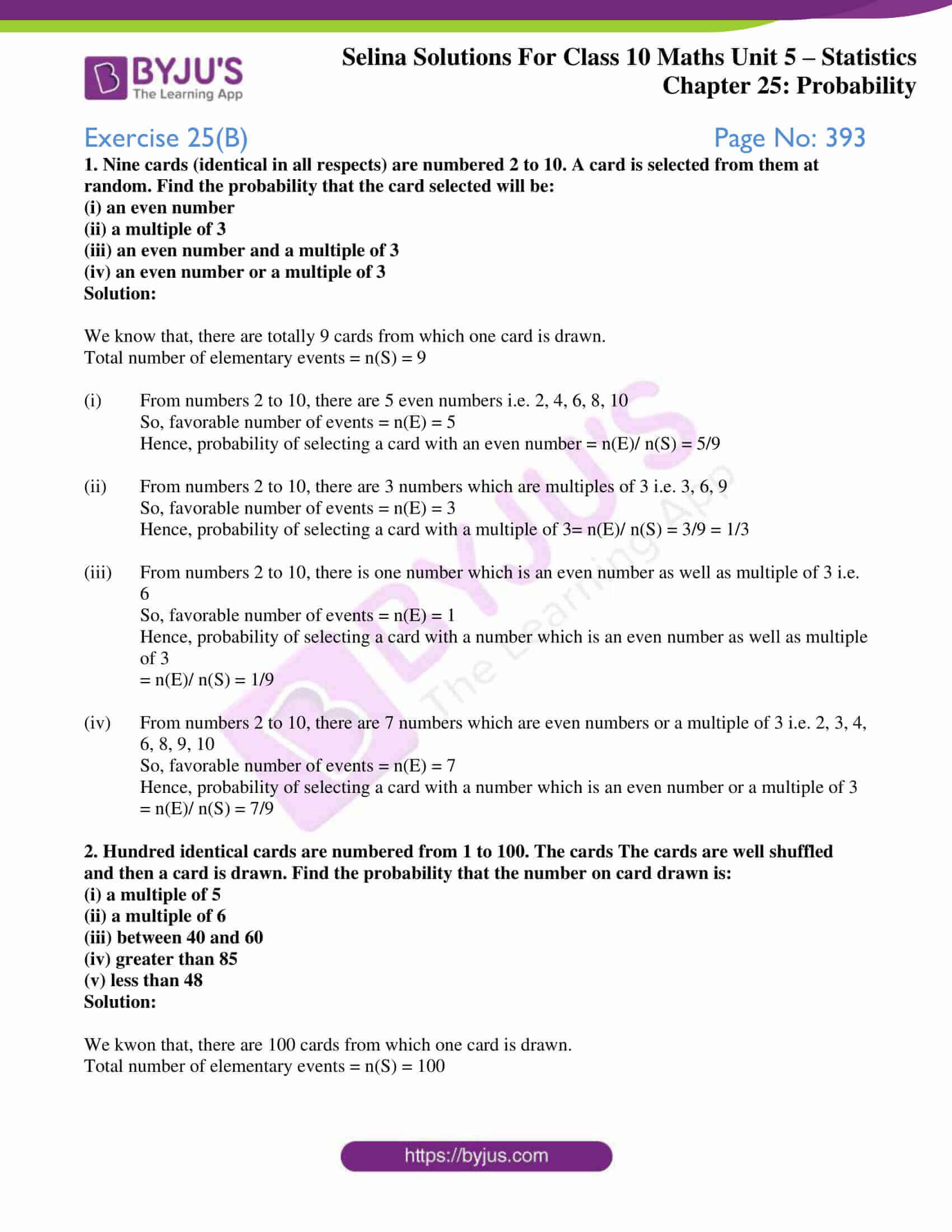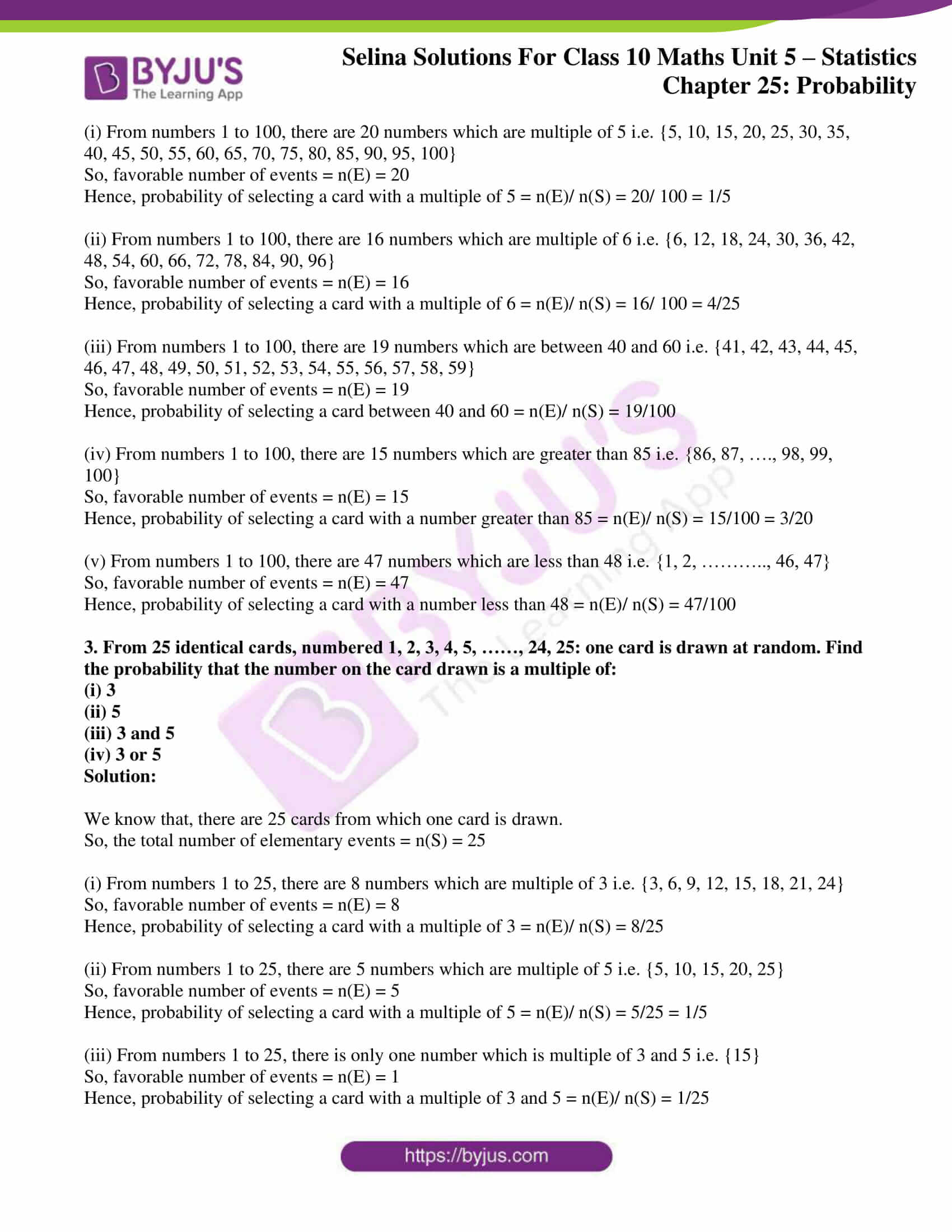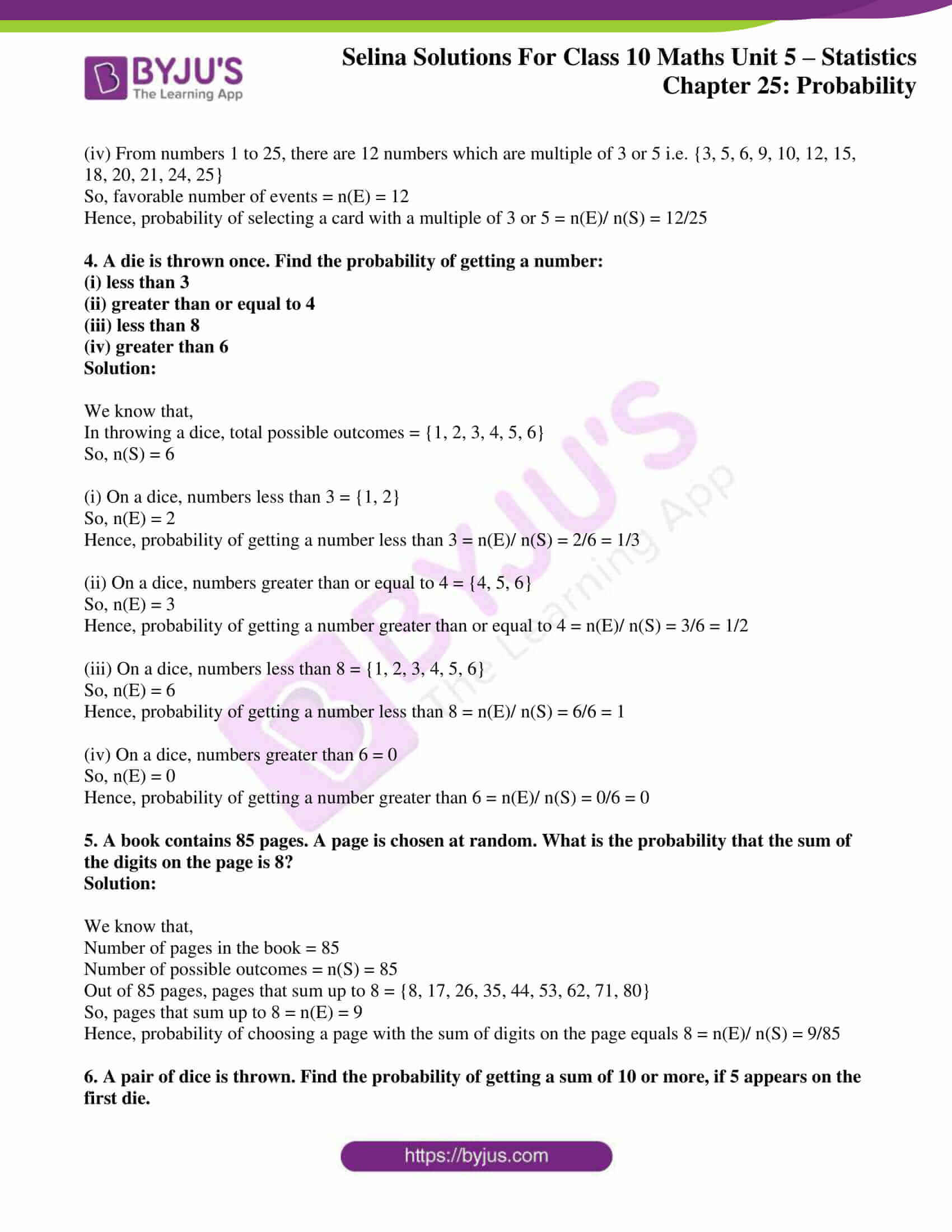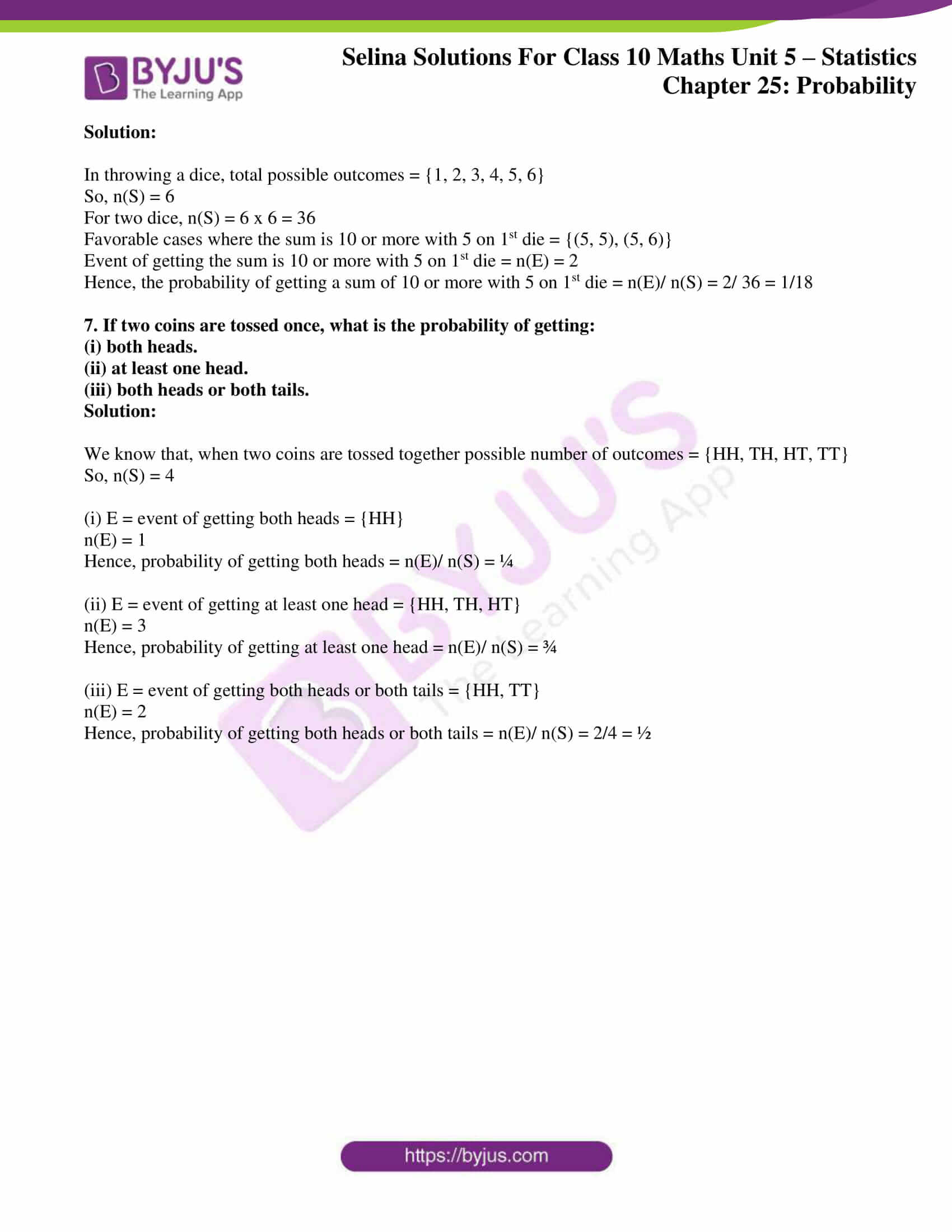# Selina Solutions Concise Maths Class 10 Chapter 25 Probability Exercise 25(B)

Events like tossing a coin once and several times, throwing a dice or two dice simultaneously and picking cards from a pack are the major topics covered in this exercise. The Selina Solutions for Class 10 Maths is the best resource a student can have for doing quick reference and preparations for exams. The Concise Selina Solutions for Class 10 Maths Chapter 25 Probability Exercise 25(B) solutions are available in a PDF in the below link.

## Selina Solutions Concise Maths Class 10 Chapter 25 Probability Exercise 25(B) Download PDF### Access Selina Solutions Concise Maths Class 10 Chapter 25 Probability Exercise 25(B)

1. Nine cards (identical in all respects) are numbered 2 to 10. A card is selected from them at random. Find the probability that the card selected will be:

(i) an even number

(ii) a multiple of 3

(iii) an even number and a multiple of 3

(iv) an even number or a multiple of 3

Solution:

We know that, there are totally 9 cards from which one card is drawn.

Total number of elementary events = n(S) = 9

(i) From numbers 2 to 10, there are 5 even numbers i.e. 2, 4, 6, 8, 10

So, favorable number of events = n(E) = 5

Hence, probability of selecting a card with an even number = n(E)/ n(S) = 5/9

(ii) From numbers 2 to 10, there are 3 numbers which are multiples of 3 i.e. 3, 6, 9

So, favorable number of events = n(E) = 3

Hence, probability of selecting a card with a multiple of 3= n(E)/ n(S) = 3/9 = 1/3

(iii) From numbers 2 to 10, there is one number which is an even number as well as multiple of 3 i.e. 6

So, favorable number of events = n(E) = 1

Hence, probability of selecting a card with a number which is an even number as well as multiple of 3

= n(E)/ n(S) = 1/9

(iv) From numbers 2 to 10, there are 7 numbers which are even numbers or a multiple of 3 i.e. 2, 3, 4, 6, 8, 9, 10

So, favorable number of events = n(E) = 7

Hence, probability of selecting a card with a number which is an even number or a multiple of 3

= n(E)/ n(S) = 7/9

2. Hundred identical cards are numbered from 1 to 100. The cards The cards are well shuffled and then a card is drawn. Find the probability that the number on card drawn is:

(i) a multiple of 5

(ii) a multiple of 6

(iii) between 40 and 60

(iv) greater than 85

(v) less than 48

Solution:

We kwon that, there are 100 cards from which one card is drawn.

Total number of elementary events = n(S) = 100

(i) From numbers 1 to 100, there are 20 numbers which are multiple of 5 i.e. {5, 10, 15, 20, 25, 30, 35, 40, 45, 50, 55, 60, 65, 70, 75, 80, 85, 90, 95, 100}

So, favorable number of events = n(E) = 20

Hence, probability of selecting a card with a multiple of 5 = n(E)/ n(S) = 20/ 100 = 1/5

(ii) From numbers 1 to 100, there are 16 numbers which are multiple of 6 i.e. {6, 12, 18, 24, 30, 36, 42, 48, 54, 60, 66, 72, 78, 84, 90, 96}

So, favorable number of events = n(E) = 16

Hence, probability of selecting a card with a multiple of 6 = n(E)/ n(S) = 16/ 100 = 4/25

(iii) From numbers 1 to 100, there are 19 numbers which are between 40 and 60 i.e. {41, 42, 43, 44, 45, 46, 47, 48, 49, 50, 51, 52, 53, 54, 55, 56, 57, 58, 59}

So, favorable number of events = n(E) = 19

Hence, probability of selecting a card between 40 and 60 = n(E)/ n(S) = 19/100

(iv) From numbers 1 to 100, there are 15 numbers which are greater than 85 i.e. {86, 87, …., 98, 99, 100}

So, favorable number of events = n(E) = 15

Hence, probability of selecting a card with a number greater than 85 = n(E)/ n(S) = 15/100 = 3/20

(v) From numbers 1 to 100, there are 47 numbers which are less than 48 i.e. {1, 2, ……….., 46, 47}

So, favorable number of events = n(E) = 47

Hence, probability of selecting a card with a number less than 48 = n(E)/ n(S) = 47/100

3. From 25 identical cards, numbered 1, 2, 3, 4, 5, ……, 24, 25: one card is drawn at random. Find the probability that the number on the card drawn is a multiple of:

(i) 3

(ii) 5

(iii) 3 and 5

(iv) 3 or 5

Solution:

We know that, there are 25 cards from which one card is drawn.

So, the total number of elementary events = n(S) = 25

(i) From numbers 1 to 25, there are 8 numbers which are multiple of 3 i.e. {3, 6, 9, 12, 15, 18, 21, 24}

So, favorable number of events = n(E) = 8

Hence, probability of selecting a card with a multiple of 3 = n(E)/ n(S) = 8/25

(ii) From numbers 1 to 25, there are 5 numbers which are multiple of 5 i.e. {5, 10, 15, 20, 25}

So, favorable number of events = n(E) = 5

Hence, probability of selecting a card with a multiple of 5 = n(E)/ n(S) = 5/25 = 1/5

(iii) From numbers 1 to 25, there is only one number which is multiple of 3 and 5 i.e. {15}

So, favorable number of events = n(E) = 1

Hence, probability of selecting a card with a multiple of 3 and 5 = n(E)/ n(S) = 1/25

(iv) From numbers 1 to 25, there are 12 numbers which are multiple of 3 or 5 i.e. {3, 5, 6, 9, 10, 12, 15, 18, 20, 21, 24, 25}

So, favorable number of events = n(E) = 12

Hence, probability of selecting a card with a multiple of 3 or 5 = n(E)/ n(S) = 12/25

4. A die is thrown once. Find the probability of getting a number:

(i) less than 3

(ii) greater than or equal to 4

(iii) less than 8

(iv) greater than 6

Solution:

We know that,

In throwing a dice, total possible outcomes = {1, 2, 3, 4, 5, 6}

So, n(S) = 6

(i) On a dice, numbers less than 3 = {1, 2}

So, n(E) = 2

Hence, probability of getting a number less than 3 = n(E)/ n(S) = 2/6 = 1/3

(ii) On a dice, numbers greater than or equal to 4 = {4, 5, 6}

So, n(E) = 3

Hence, probability of getting a number greater than or equal to 4 = n(E)/ n(S) = 3/6 = 1/2

(iii) On a dice, numbers less than 8 = {1, 2, 3, 4, 5, 6}

So, n(E) = 6

Hence, probability of getting a number less than 8 = n(E)/ n(S) = 6/6 = 1

(iv) On a dice, numbers greater than 6 = 0

So, n(E) = 0

Hence, probability of getting a number greater than 6 = n(E)/ n(S) = 0/6 = 0

5. A book contains 85 pages. A page is chosen at random. What is the probability that the sum of the digits on the page is 8?

Solution:

We know that,

Number of pages in the book = 85

Number of possible outcomes = n(S) = 85

Out of 85 pages, pages that sum up to 8 = {8, 17, 26, 35, 44, 53, 62, 71, 80}

So, pages that sum up to 8 = n(E) = 9

Hence, probability of choosing a page with the sum of digits on the page equals 8 = n(E)/ n(S) = 9/85

6. A pair of dice is thrown. Find the probability of getting a sum of 10 or more, if 5 appears on the first die.

Solution:

In throwing a dice, total possible outcomes = {1, 2, 3, 4, 5, 6}

So, n(S) = 6

For two dice, n(S) = 6 x 6 = 36

Favorable cases where the sum is 10 or more with 5 on 1st die = {(5, 5), (5, 6)}

Event of getting the sum is 10 or more with 5 on 1st die = n(E) = 2

Hence, the probability of getting a sum of 10 or more with 5 on 1st die = n(E)/ n(S) = 2/ 36 = 1/18

7. If two coins are tossed once, what is the probability of getting:

(iii) both heads or both tails.

Solution:

We know that, when two coins are tossed together possible number of outcomes = {HH, TH, HT, TT}

So, n(S) = 4

(i) E = event of getting both heads = {HH}

n(E) = 1

Hence, probability of getting both heads = n(E)/ n(S) = ¼

(ii) E = event of getting at least one head = {HH, TH, HT}

n(E) = 3

Hence, probability of getting at least one head = n(E)/ n(S) = ¾

(iii) E = event of getting both heads or both tails = {HH, TT}

n(E) = 2

Hence, probability of getting both heads or both tails = n(E)/ n(S) = 2/4 = ½